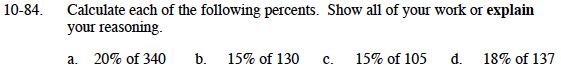### Home > MC1 > Chapter 10 > Lesson 10.2.2 > Problem10-84

10-84.10% of 340 is 34
20% = 2(10%)

Try following the steps in parts (a) and (b).

10% of 130 is 13

10% of 137 is 13.7
1% of 137 is 1.37

18% = 2(10%) − 2(1%)
18% of 137 = 2(13.7) − 2(1.37)
= 27.4 − 2.74
= 24.66